# Class 11 Maths NCERT Solutions for Chapter 8 Binomial Theorem Miscellaneous Exercise### Binomial Theorem Exercise Miscellaneous Solutions

1. Find a, b and n in the expansion of (a + b)n if the first three terms of the expansion are 729, 7290 and 30375, respectively.

Solution

It is known that (+ 1)th term, (Tr+1), in the binomial expansion of (b)n is given by
Tr+1 = nCr an-r br .
The first three terms of the expansion are given as 729, 7290, and 30375 respectively.
Therefore, we obtain
T1 = nC0 an-0 b0 = an = 729  ...(1)
T2 = nC1 an-1 b1 = nan-1 b = 7290  ...(2)
T3 = nC2 an-2 b= [n(n-1)/2] an-2 b2  = 30375 ...(3)
Dividing (2) by (1), we obtain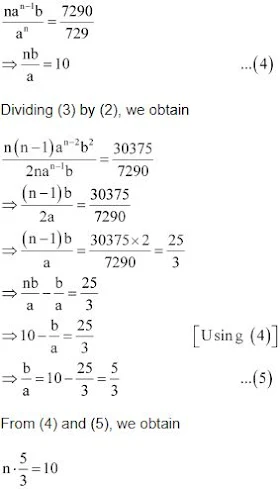⇒ n = 6
Substituting n = 6 in equation (1), we obtain
a6 = 729
⇒ a = 6√729 = 3
From (5), we obtain
b/3 = 5/3 ⇒ 5
Thus, a = 3 , b = 5 , and n = 6.

2. Find a if the coefficients of x2 and x3 in the expansion of (3 + ax)9 are equal.

Solution

It is known that (+ 1)th term, (Tr+1), in the binomial expansion of (b)n is given by Tr+1 = nCr an-r br ..
Assuming that x2 occurs in the (r + 1)th term in the expansion of (3 + ax)9, we obtain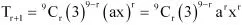Comparing the indices of x in x2 and in Tr + 1, we obtain
r = 2
Thus, the coefficient of x2 is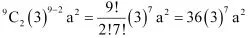Assuming that x3 occurs in the (k + 1)th term in the expansion of (3 +ax)9 , we obtain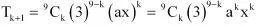Comparing the indices of x in x3 and in Tk+1 , we obtain
k = 3
Thus, the coefficient of x3 is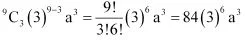It is given that the coefficients of x2 and x3 are the same.
84(3)6 a3 = 36(3)7 a2
⇒ 84a = 36× 3
⇒ a = (36× 3)/84 = 104/84
⇒ a = 9/7
Thus, the required value of a is 9/7.

3. Find the coefficient of x5 in the product (1 + 2x)6 (1 – x)7 using binomial theorem.

Solution

Using Binomial Theorem, the expressions, (1 + 2x)6 and (1 – x)7, can be expanded as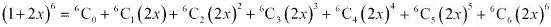= 1 + 6(2x) + 15(2x)2 + 20(2x)3 + 15(2x)4 + 6(2x)5 + (2x)6
= 1 + 12x + 60x2 + 160x3 + 240x4 + 192x5 + 64x6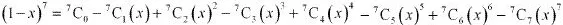= 1 -7x + 21x2 - 35x3 + 35x4 - 21x5 + 7x6 - x7
∴ (1 + 2x)6 (1 -x)7
= (1 + 12x + 60x2 + 160x3 + 240x4 + 192x5 + 64x6 )(1 - 7x + 21x2 - 35x3 + 35x4 - 21x5 + 7x6 - x7)
The complete multiplication of the two brackets is not required to be carried out.
Only those terms, which involve x5 , are required.
The terms containing x5 are
1(-21x5) + (12x)(35x4) + (60x2)(-35x3) + (160x3 )(21x2) + (240x4)(-7x) + (192x5)(1)
= 171x5
Thus, the coefficient of x5 in the given product is  171.

4. If a and b are distinct integers, prove that a – b is a factor of an – bn, whenever n is a positive integer.
[Hint: write an = (a – b + b)n and expand]

Solution

In order to prove that (a – b) is a factor of (an – bn), it has to be proved that
an – bn = k (a – b), where k is some natural number
It can be written that, a = a – b + b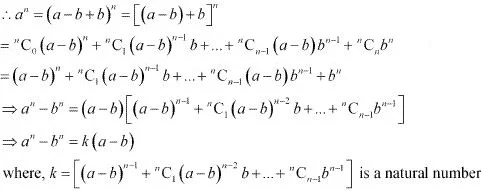This shows that (a - b) is a factor of (an – bn), where n is a positive integer.

5. Evaluate (√3 + √2)6  - (√3 - √2)6

Solution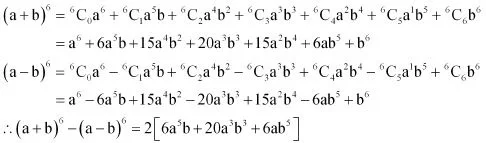Putting a = √3 and b = √2, we obtain
(√3 + √2)6  - (√3 - √2)6  = 2[6(√3)5 (√2) + 20(√3)3 (√2)3  + 6(√3)(√2)5]
= 2[54√6 + 120√6 + 24√6]
= 2 × 198√6
= 396√6

6. Find the value of [a2 + √(a2 - 1)]4 + [a2 - √(a2 - 1)]4

Solution

Firstly, the expression (x + y)4 + (x – y)4 is simplified by using Binomial Theorem.
This can be done asPutting x = a2 and y = √(a2 - 1) , we obtain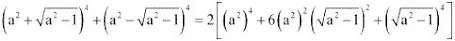= 2[a8 + 6a4 (a2 -1) + (a2 -1)2 ]
= 2[a8 + 6a6 - 6a4 + a4 - 2a2 + 1]
= 2[a8 + 6a6 - 5a4 - 2a2 + 1]
= 2a8 + 12a6 - 10a4 - 4a2 + 2

7. Find an approximation of (0.99)5 using the first three terms of its expansion.

Solution

0.99 = 1 - 0.01
∴ (0.99)5 = (1 - 0.01)5
5C0 (1)5 - 5C1 (1)4 (0.01) + 5C2 (1)3 (0.01)2  (Approximately)
= 1 - 5(0.01) + 10(0.01)2
1 - 0.05 + 0.001
= 1.001 - 0.05
= 0.951
Thus, the value of (0.99)5 is approximately 0.951.

8. Find n, if the ratio of the fifth term from the beginning to the fifth term from the end in the expansion of (4√2 + 1/4√3 )n is √6 : 1

Solution

(a + b)n = nC0an + nC1an-1b + nC2an-2b2 + ... + nCn-1 abn-1 + nCnbn,
Fifth term from the beginning = nC4 an-4 b4
Fifth term from the end = nCn-4 a4 bn-4
Therefore, it is evident that in the expansion of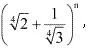the fifth term from the beginning is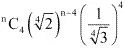and the fifth term from the end is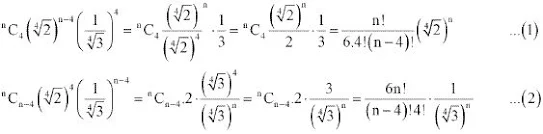It is given that the ratio of the fifth term from the beginning to the fifth term from the end is √6 : 1. Therefore, from (1) and (2), we obtain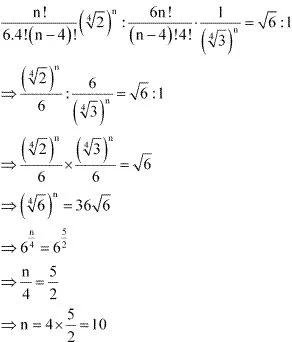Thus, the value of n is 10.

9. Expand using Binomial Theorem (1 + x/2 - 2/x)4, x ≠ 0

Solution

Using Binomial Theorem, the given expression (1 + x/2 - 2/x)4 can be expanded as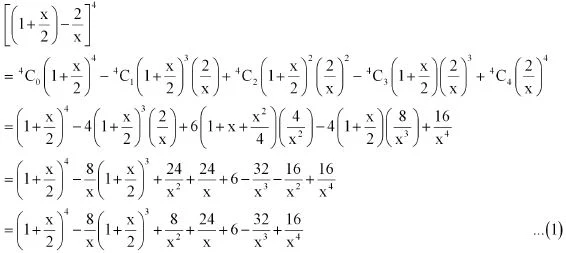Again by using Binomial Theorem, we obtain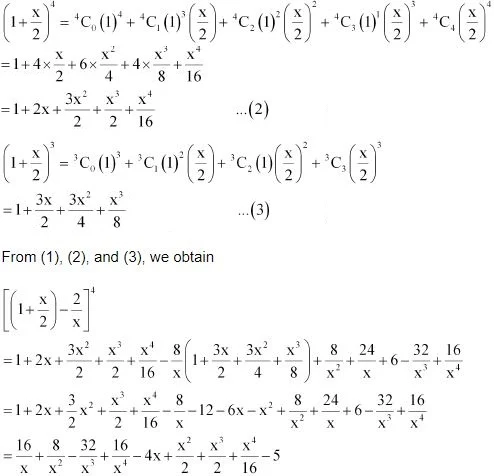10. Find the expansion of (3x2 - 2ax + 3a2)3 using binomial theorem.

Solution

Using Binomial Theorem, the given expression (3x2 - 2ax + 3a2)3 can be expanded as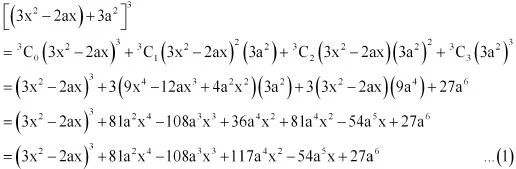Again by using Binomial Theorem, we obtain
(3x2 - 2ax)3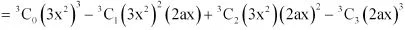= 27x6 - 3(9x4 )(2ax) + 3(3x2 )(4a2 x2 ) - 8a3x3
= 27x6 - 54ax5 + 36a2 x4 - 8a3 x3 ...(2)
From (1) and (2), we obtain
(3x2 - 2ax + 3a2)3
= 27x6 - 54ax5 + 36a2 x4 - 8a3 x3 + 81a2 x4 - 108a3 x3 + 117a4 x2 - 54a5 x + 27a6
= 27x6 - 54ax5 + 117a2 x4 - 116a3 x3 + 117a4 x2 - 54a5 x + 27a6